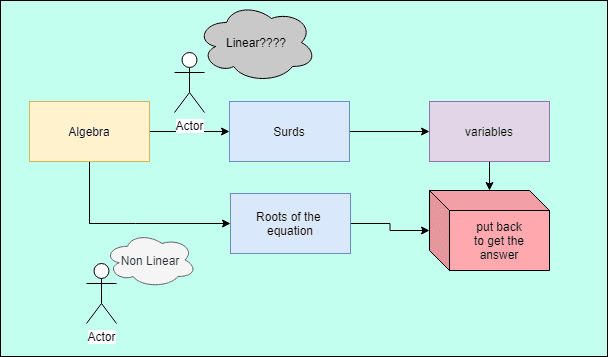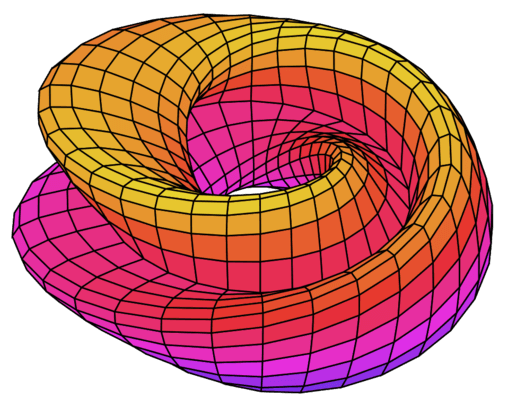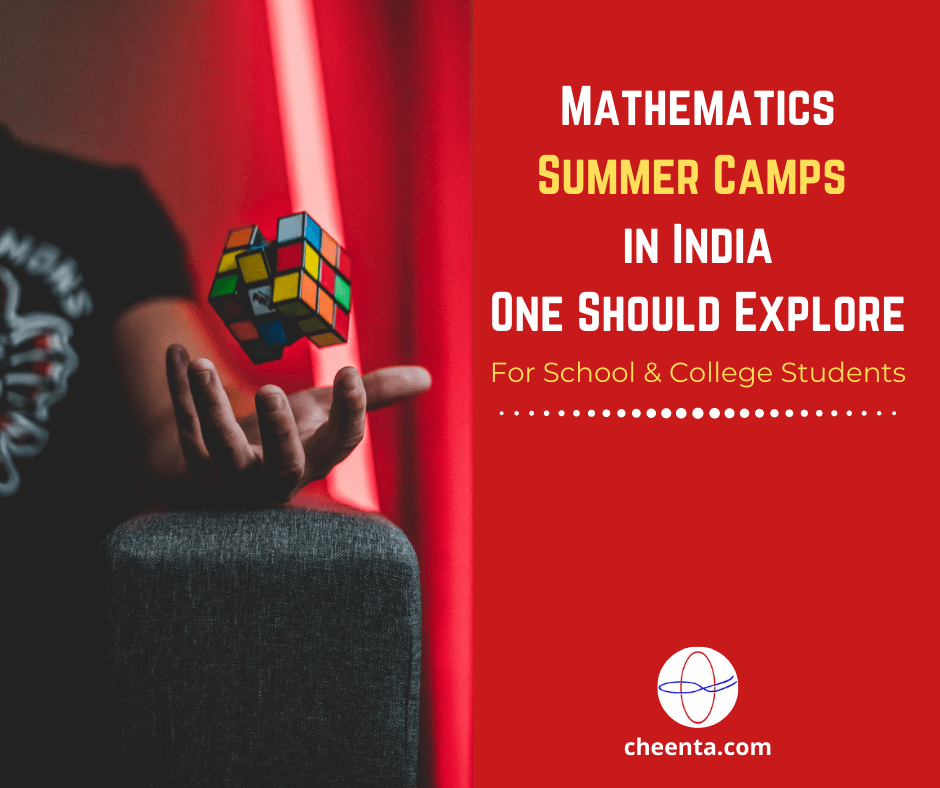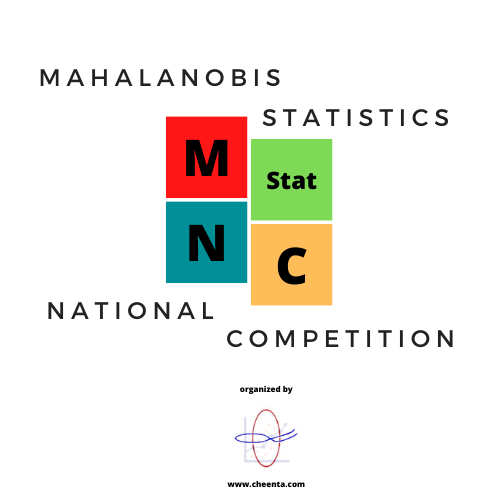Categories

# Root of Equation- B.Stat. (Hons.) Admission Test 2005 – Objective Problem 2

Try this beautiful problem of Algebra prticularly in cubic equation fromB.Stat. (Hons.) Admission Test 2005. You may use sequential hints to help you solve the problem.

## Competency in Focus: Root of Equation

This problem from Root of equation for B.Stat. (Hons.) Admission Test 2005 Objective Problem 2  is based on calculating a variable in a given equation.

## First look at the knowledge graph:-## Next understand the problem

If $\sqrt{3}+1$ is a root of the equation $3x^{3}+ax^{2}+bx+12=0$ where a and b are rational numbers, then b is equal to (A) -6 (B) 2 (C) 6 (D) 10

### Algebra (Root of equation)

4/10
##### Suggested Book

Challenges and Thrills in Pre College Mathematics Excursion Of Mathematics

Do you really need a hint? Try it first!
If the co effeicent of any polynomial equation is rational number and one of the root is a surd or coplex number, then the other root must be the conjugate.
Since our polynomial is of degree 3, there must be three roots of the equation. We already know the two of them so let the third one is $\gamma$.
we can use Vieta’s Theorem, that gives, the product of the roots is $\frac{c}{a}$ in our case $\frac{-12}{3}$ =$-4$. so we can say that $(1+\sqrt{3}) \times(1-\sqrt{3}) \times \gamma=-4$
Now we have calculated the value of gamma. Also we have from Vieta’s Theorem, that sum of products of the roots taken two roots at a time is $\frac{b}{3}$. So we can write,   $\frac{b}{3}=(1+\sqrt{3}) \times(1-\sqrt{3})+_{\gamma} \times\{(1+\sqrt{3})+(1-\sqrt{3})\}=2$.

## I.S.I. & C.M.I. Program

Indian Statistical Institute and Chennai Mathematical Institute offer challenging bachelor’s program for gifted students. These courses are: B.Stat and B.Math program in I.S.I., B.Sc. Math in C.M.I.
The entrances to these programs are far more challenging than usual engineering entrances. Cheenta offers an intense, problem-driven program for these two entrances.## IIT JAM Stat Mock Test Toppers

We are really happy with the performance of our students and thus, we have initiated to name the Toppers of IIT JAM Stat Mock Test. These toppers are named in this leader board according to their performance in IIT JAM Stat Mock Tests. So, here goes the list: Mock...

## Pigeonhole Principle

“The Pigeonhole principle” ~ Students who have never heard may think that it is a joke. Pigeonhole Principle is one of the simplest but most useful ideas in mathematics. Let’s learn the Pigeonhole Principle with some applications. Pigeonhole Principle Definition: In...## Mathematics Summer Camps in India One Should Explore

Mathematics Summer Camps help students to feel the richness of Mathematics. These summer mathematics programme in India instills the love for Mathematics in students. In this post, we are going to discuss the Mathematics Summer Camps in India for School and College...

## How to use Vectors and Carpet Theorem in Geometry 1?

Here is a video solution for a Problem based on using Vectors and Carpet Theorem in Geometry 1? This problem is helpful for Math Olympiad, ISI & CMI Entrance, and other math contests. Watch and Learn! Here goes the question… Given ABCD is a quadrilateral and P and...## Mahalanobis National Statistics Competition

Mahalanobis National Statistics Competition = MNStatC organized by Cheenta Statistics Department with exciting cash prizes. What is MNStatC? Mahalanobis National Statistics Competition (MNStatC) is a national level statistics competition, aimed at undergraduate...

## Carpet Strategy in Geometry | Watch and Learn

Here is a video solution for a Problem based on Carpet Strategy in Geometry. This problem is helpful for Math Olympiad, ISI & CMI Entrance, and other math contests. Watch and Learn! Here goes the question… Suppose ABCD is a square and X is a point on BC such that...## Bijection Principle Problem | ISI Entrance TOMATO Obj 22

Here is a video solution for a Problem based on Bijection Principle. This is an Objective question 22 from TOMATO for ISI Entrance. Watch and Learn! Here goes the question… Given that: x+y+z=10, where x, y and z are natural numbers. How many such solutions are...## What is the Area of Quadrilateral? | AMC 12 2018 | Problem 13

Here is a video solution for a Problem based on finding the area of a quadrilateral. This question is from American Mathematics Competition, AMC 12, 2018. Watch and Learn! Here goes the question… Connect the centroids of the four triangles in a square. Can you find...## Solving Weird Equations using Inequality | TOMATO Problem 78

Here is a video solution for ISI Entrance Number Theory Problems based on solving weird equations using Inequality. Watch and Learn! Here goes the question… Solve: 2 \cos ^{2}\left(x^{3}+x\right)=2^{x}+2^{-x} We will recommend you to try the problem yourself. Done?...

## AM-GM Inequality Problem | ISI Entrance

Here is a video solution for ISI Entrance Number Theory Problems based on AM-GM Inequality Problem. Watch and Learn! Here goes the question… a, b, c, d are positive real numbers. Prove that: (1+a)(1+b)(1+c)(1+d) <= 16. We will recommend you to try the problem...

This site uses Akismet to reduce spam. Learn how your comment data is processed.